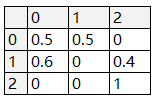# 利用状态转移矩阵和VBA求游戏中各种事件达成次数的期望

``````举个例子：

+0强化至+1，成功率50%，失败后等级不变。
+1强化至+2，成功率40%，失败后等级下降至+0。该事件的一步状态转移矩阵
``````第一列第一行的0.5代表，强化一次从状态+0变为状态+2的概率为0.5,

``````矩阵的乘法规则自己百度。该事件的二步状态转移矩阵
``````第i列第j行的元素即代表强化二次从状态i变为状态j的概率。

``````Sub Matrix()
Dim i, j, k, a(1 To 10000, 1 To 10, 1 To 10), b(1 To 10000, 1 To 10, 1 To 10), p(1 To 1000), e(1 To 1000)

For i = 1 To 3              '一步转移矩阵写入
For j = 1 To 3
a(1, i, j) = Cells(i + 3, j + 2)
Next j
Next i

p(1) = 0

For k = 2 To 1000            'K步转移矩阵计算
For i = 1 To 3
For j = 1 To 3

a(k, i, j) = a(1, i, 1) * a(k - 1, 1, j) + a(1, i, 2) * a(k - 1, 2, j) + a(1, i, 3) * a(k - 1, 3, j)

Next j
Next i
p(k) = a(k, 1, 3)            'Pk=[K步状态转移矩阵中的Pij]
Next k

e(1) = 0

For k = 2 To 1000
e(k) = e(k - 1) + k * (p(k) - p(k - 1))     '累加求和求得最终期望值
Next k

MsgBox e(1000)

End Sub``````

## 我发现了一种更简单的办法

mathematica中有内置的离散马科夫过程函数，可以进行快捷的计算： 第一步：列出一步状态转移矩阵 第二步：在Mathematica中读取一步状态转移矩阵
``````xlsxPath= "C:\Users\Administrator\Desktop\一步状态转移矩阵.xlsx";

m =Import[xlsxPath, "Data"][][[2 ;; 4, 2 ;; 4]]``````

``````markovProcess = DiscreteMarkovProcess[1,m];
Mean[FirstPassageTimeDistribution[markovProcess,3]]``````